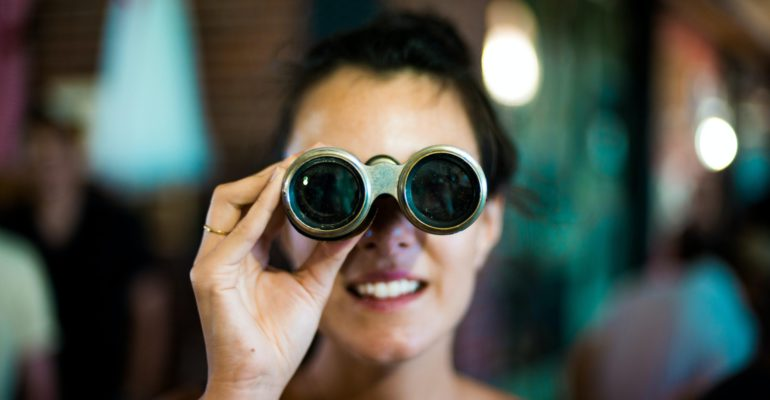Thanks to theidioms.com

## Open-Source Computer Vision Projects (With Tutorials)# Open-Source Computer Vision Projects (With Tutorials)

The computer vision projects listed below are categorized in an experience-wise manner. All of these projects can be implemented using Python.

#### Beginner-friendlyComputer Vision Data Science Projects

1. Face and Eyes Detection using Haar Cascades Github Link, Video Tutorial, Written Tutorial

Face and Eyes Detection is a project that takes in a video image frame as an input and outputs the location of the eyes and face (in x-y coordinates) in that image frame. The script is fairly easy to understand and uses Haar Cascades for detecting the face and the eyes if found in the image frame.

Full code for Face and Eyes Detection using Haar Cascades:

```import numpy as np
import cv2

cap = cv2.VideoCapture(0)

while 1:
gray = cv2.cvtColor(img, cv2.COLOR_BGR2GRAY)

for (x,y,w,h) in faces:
cv2.rectangle(img,(x,y),(x+w,y+h),(255,0,0),2)
roi_gray = gray[y:y+h, x:x+w]
roi_color = img[y:y+h, x:x+w]

for (ex,ey,ew,eh) in eyes:
cv2.rectangle(roi_color,(ex,ey),(ex+ew,ey+eh),(0,255,0),2)

cv2.imshow('img',img)
k = cv2.waitKey(30) & 0xff
if k == 27:
break

cap.release()
cv2.destroyAllWindows()```

2. Template MatchingVideo Tutorial, Written Tutorial

Template matching is a project that takes in a template image and matches it with an image frame and is used in digital image processing for finding small parts of an image which match a template image.

Full code for Template Matching:

```import cv2
import numpy as np

# Convert color to grayscale
img_gray = cv2.cvtColor(img_rgb, cv2.COLOR_BGR2GRAY)

w, h = template.shape[::-1]

res = cv2.matchTemplate(img_gray,template,cv2.TM_CCOEFF_NORMED)
threshold = 0.8
loc = np.where( res >= threshold)

for pt in zip(*loc[::-1]):
cv2.rectangle(img_rgb, pt, (pt + w, pt + h), (0,255,255), 2)

cv2.imshow('Detected',img_rgb)```

3. Foreground Extraction using grabCut – Video Tutorial, Written Tutorial

Foreground Extraction using grabCut is a project that extracts the foreground element from an image frame. This foreground extraction tutorial by Sentdex uses his own image as a test image for the tutorial but you can do it with an image that is suitable for you.

Full code for Foreground Extraction using grabCut:

```import numpy as np
import cv2
from matplotlib import pyplot as plt

bgdModel = np.zeros((1,65),np.float64)
fgdModel = np.zeros((1,65),np.float64)

rect = (161,79,150,150)

plt.imshow(img)
plt.colorbar()
plt.show()```

4. Optical Character Recognition using Tesseract – Video Tutorial

Optical Character Recognition is a project in which text written in an image is extracted and converted into plain text form.

Full code for Optical Character Recognition using Tesseract:

```from PIL import Image
import pytesseract

# Replace test.png with your image name

img = Image.open("test.png")
text = pytesseract.image_to_string(img, lang="en")
print(text)```

#### Intermediate Computer Vision Data Science Projects

1. Digit Recognition using Deep Learning – Video Tutorial, Written Tutorial

Digit Recognition is the task of recognizing the value displayed in an image frame using Deep Learning. This digit recognition tutorial by Sentdex uses Python, TensorFlow and Keras to predict the numbers written in the MNIST image dataset.

2. Object Detection using Deep Learning Video Tutorial, Written Tutorial

Object Detection is the task of recognizing objects on an image frame based on a reference image on which a deep learning model is trained on. This object detection tutorial by Sentdex uses Python and TensorFlow for detecting food items in images.

Face Recognition is a computer vision task of recognizing the faces of people in an image frame. At first, face detection is carried out to find if an image frame has human faces in it or not and then, a face recognition algorithm is used to match detected faces with known faces from an image database.

Watch the full tutorial by TraversyMedia to learn about it in detail:

4. License Plate Detection and Recognition – Video Tutorial

License Plate Detection and Recognition is a project that uses detection and OCR techniques to find the number written in a vehicle’s license plate.

#### Expert Computer Vision Data Science Projects

1. Gaming Artificial IntelligenceGitHub Link, Video Tutorial

Gaming Artificial Intelligence or Gaming AI is a type of AI that is able to play computer games on its own. This tutorial by Sentdex covers such kind of AI in a detailed fashion implementing various Deep Learning algorithms for achieving various tasks.

2. Lane finder for Self Driving Cars – Video Tutorial

Lane Finder for Self Driving Cars is a project that uses Python and Computer Vision to find lanes in a video containing the movement of a car. The algorithm can be used to find lane lines accurately on an image.

#### In Conclusion

What do you think about this article on ‘Open-Source Computer Vision Projects (with Tutorials)’? Do you have any recommendation for us to include in the above list? Let us know.

Also, if you are trying to become a data scientist or are looking for your first job, this article on ‘Why is it hard to get a data science job?